# Conditional probability formula |Bayes Theorem|Total Probability Law

This Chapter we would be taking a look at the below topics
Conditional probability formula
Bayes Theorem
Total Probability Law
Before going into these topics,let revise and flashback our memory for the things we know on Probability.

## Flashback from Class 11

### What is Probability

Probability is the measure of the likeliness that an event will occur. Probability is quantified as a number between 0 and 1 (where 0 indicates impossibility and 1 indicates certainty)

### Terms related to Probability

Randomness or Random experiments
When we perform experiments in science and engineering repeatedly under very nearly identical conditions, we almost get the same result. Such experiments are called deterministic experiments. There also exists experiments When next outcome of the experiment cannot be determined even they are performed under nearly identical conditions then we say it is a random experiment

e.g. Consider the dice. When we throw the dice, we cannot determine what number will come Since we cannot predict the next outcome, we may say it is a random experiment

Probability theory is concerned with such random phenomena or random experiments

### Trial

A trial is an action which results in one or several outcomes, for example each toss of the coin and each throw of the die are called trials

Independent Trial
Successive trials of some random event for example tosses of a coin, throws of a die are said to be independent if the outcome of any one trial does not impact the outcomes of any others.

Sample space
It is a set of all possible outcomes of a random experiment which we defined earlier

e.g. when we coin is tossed, the possible outcome are Head and Tail. So sample space is Head and tail
It is generally denoted by letter S

The sample space can be either be finite or infinite.

Example for infinite Sample space would be an experiment where we are tossing the coin    until Head comes up. Sample space {(H), (TH), (TTH), (TTTH)….}

We can use Tree diagram to find out the sample space. Permutation and combination studied in earlier chapter also will be used extensively to find the sample space
Event
An event is a possible outcome of the Experiment. Any subset E of the sample space S is called an event.

Example of events

1. Roll a die: the outcome is even {2, 4, 6}.

2. Flip a coin twice and the two results are different:

{(H, T), (T, H)}.

Different type of events
 Elementary or simple event If it contains the single elements of the sample space Compound event If it contains more than single elements of the sample space Impossible event If it can never occur. Sure or certain event An event which is sure to occur

### Algebra of events operations

We know the event are sets and they are subset of Sample space. So normal set operations can be done over them also

Let A and B are two events of the Sample Space S
 Union: (A ∪ B) an outcome is in A ∪ B if it is either in A or in B.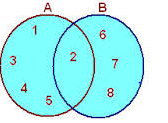Intersection: (A ∩ B), AB: an outcome is in AB if it is in both A and B.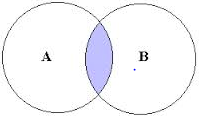Difference (A -B), the event of occurrence of A but not B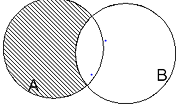Difference (B -A), the event of occurrence of B but not A AC  : The event of non-occurrence of the event A.It is also called the complementary event of A

Some Important Definition

Equally Likely Outcomes

The outcome of the random experiments are called equally likely outcomes. If all of them are equal preferences.

Exhaustive Outcomes

The outcome of the random experiments are called exhaustive outcomes. If they cover all the possible outcomes of the experiments

### Classical Probability

The theoretical probability or the classical probability of the event A in a random experiment having exhaustive, equal likely outcome is defined as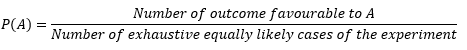1) Let Number of exhaustive equally likely cases of the experiment =n

Number of outcome favorable to A=m

Then

P( A) = m/n

Also you may have observed that

0≤ m ≤ n

Or

0 ≤ m/n ≤ 1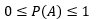2) The event ?, representing ‘not A’, is called the complement of the event A. We also say that ? and A are complementary events.

Now

P(?) = (n-m)/n = 1 - (m/n) = 1- P(A)

Or

P(A) +P(?)=1

4) The probability of an event (U) which is impossible to occur is 0. Such an event is called an impossible event

P(U)=0

5) The probability of an event (X) which is sure (or certain) to occur is 1. Such an event is called a sure event or a certain event

P(X)=1

5) Probability of any event can be as6) Odds in favor of the happening of the event is defined P(A) and P(?)

and P( ?)

Let it be    m:n

Then   P(A)/ P( ?)  m/n

Or

P(A)/(1- P( A))=  m/n

Or

P(A) = m/m+n

7) Odds against the happening of the event is defined as ratio of  P( ?) and  P(A)

Let it be    m:n

Then

P(A)  =n/m+n

How to Solve Probability questions

1)  Find out the number of elements in the sample space S of the given random experiment. It would be nice to write sample space elements also if possible

2) Find out the elements which lies in the event (A) which we are looking to find probability. Count the elements in it

3)  Use classical definition of probability to find out the answe

1) Let E and F are two events of a random experiments
Then P(E ∪ F) = P(E) + P(F) − P(E   F)
If event E and F are mutually exclusive
n( E F)  = 0
Then
P(E ∪ F) = P(E) + P(F)
2)  Addition Theorem of three events
Let E, F and G are three events of a random experiments
P (E ∪ F ∪ G) = P(E) + P(F) + P(G) - P (E   F) -  P (F   G) - P (E   G ) + P (E   F G)
If event E, F and G are mutually exclusive
n(E F) = 0
n(E G) = 0
n(F G) = 0
n(E   F G)= 0
Then
P (E ∪ F ∪ G) = P(E) + P(F) + P(G)
3)
Let E and F are two events of a random experiments
Then Probability of the occurrence of the Event E   only (E Fc)
P (E Fc)  = P(E) – P (E F )
Probability of the occurrence of the Event F only ( Ec Ç F)
P (Ec F) = P(F) – P (E F)
Probability of occurrence of exactly one of the two event E and F
P[(Ec F)   ∪ (E Fc) ]  = P(E) + P(F) −2 P(E   F)

## What is Conditional Probability

Let E and F are two events of the random experiments. Probability of event A happening give the condition event F has happened is called Conditional probability
So conditional probability of E given F has happened is P(E | F).
Similarly, we can define P (F| E).
We can think these conditional probabilities in these ways also
P (E|F) -> Probability of occurrence of E given F has happened
Or
P(E|F) -> Probability of occurrence of E when event F is taken as sample space

Example
Let take an example of a random experiment of Flip a coin twice.
S = {(H, H), (H, T), (T, H), (T, T)}.
Let F be the event of first flip to be H.
And let Me be the event for two flips are not both H
Then F = {(H, H), (H, T)}, P(F) = 1/2.
E = {(H, T), (T, T), (T, H)}, P(E) = 3/4.
Now If F event occurs, in order for event E to occur, the second flip has to be T.
P(E|F) = ½

## Multiplication Theorem on Probability|Conditional probability formula

1) Let E and F are two events of a random experiments
Then P (E F) = P(E) P(F|E) if P(E) ≠0
Or
P (E   F) = P(F) P(E|F) if P(F) ≠0
Proof:
Let S be the sample space and it contains n elementary events
Let a, b, c is the number of elementary event in E, F and (E   F)
Then
P(E) = a/n
P(F) = b/n
P(E   F)= c/n
Now
P(E |F)  =c/b
P(F |E) = c/a
Now
P(E   F)= c/n
=(c/b)  (b/n)
= P(F) P( E|F) Or
P(E   F)= c/n
=(c/a)  (a/n)
= P(E) P( F|E) Hence Proved
Now
P (E F) = P(E) P(F|E) if P(E) ≠0
Or
P (E F) = P(F) P(E|F) if P(F) ≠0
Can be rewritten as
P(F|E) = P (E   F) / P(E)
Or
P(E|F) = P (E F) / P(F)
2) So far we have proved all about dependent events
What are Independent Events
Events can be "Independent", meaning each event is not affected by any other events. We can say that if the occurrence or non-occurrence of one does not affect the probability of the occurrence or non-occurrence of the other
Two events E and F are independent then
P(E|F) = P(E) and P(F|E) = P(F).
So P (E F) = P(E)P(F).
We can extend this n number of events
Also for independents events
P (E ∪ F) = P(E) + P(F) – P (E  F)
P (E ∪ F) = P(E) + P(F) – P(E) P(F)
= 1 – P(Ec) P(Fc)

## Total Probability Law

Let S be the Sample space and let F1 ,F2………Fn­    be the n mutually exclusive and exhaustive events associated with the random experiments. If E is any event which occurs with those n mutually exclusive events, then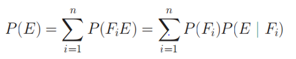## Total Probability Law Solved example

Question
I have three bags that each contain 100 marbles:
Bag A has 75 red and 25 blue marbles;
Bag B has 60 red and 40 blue marbles;
Bag C has 45 red and 55 blue marbles.
I choose one of the bags at random and then pick a marble from the chosen bag, also at random. What is the probability that the chosen marble is red?
Let E be the event that the chosen marble is red. Let Bi be the event that I choose Bag i. We already know that
P (E|BA )=0.75,
P (E|BB )=0.60,
P (E|BC )=0.45 And
P( BA) = P( BA)= P( BA) =1/3
Now the events BA ,BB ,BC   are mutually exclusive events (only one of them can happen), and secondly, because their union is the entire sample space as one the bags will be chosen for sure, i.e., P(BA ∪ BB ∪ BB)=1.
So
Using the law of total probability, we can write
P(E)   = P(E|BA) P( BA) + P(E|BB) P( BB)  + P(E|BC) P( BC) =(0.75)(1/3)+(0.60)(1/3)+(0.45)(1/3)

=0.60

## Bayes Formula

Let S be the Sample space and let F1 ,F2………Fn­    be the n mutually exclusive and exhaustive events associated with the random experiments. If E is any event which occurs with those n mutually exclusive events, then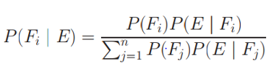Some Important points about Bayes Theorem
 Event F1  , F2……Fn They are called hypothesis P(F1) …… P(F2) These probabilities are called priori probabilities as they exist before we obtain any information from experiment P( E/F1) ……. P(E/F­n) These probabilities are called likelihood probabilities as they tell us how event A under consideration occurs P(F1/E) …. P(Fn/E) These probabilities are called the posterior probabilities as they are determined after the result of the experiment is known

## Bayes Formula Solved examples

Examples:

Question
Of all the smokers in a particular district in India   40% prefer brand X   and 60% prefer brand Y. Of those smokers who prefer brand X, 30% are females, and of those who prefer brand Y, 40% are female. What is the probability that a randomly selected smoker prefers brand A, given that the person selected is a female?
Solution
Let F1 = "prefer brand A",
F2 = "prefer brand B" and
E is the event "female".
We need to find out   P(F1|E)
Now from Bayes formulaIn this particular situation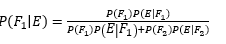So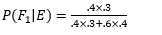Or
P(F1|E) =1/3

## Related Topics

Go back to Class 12 Main Page using below links